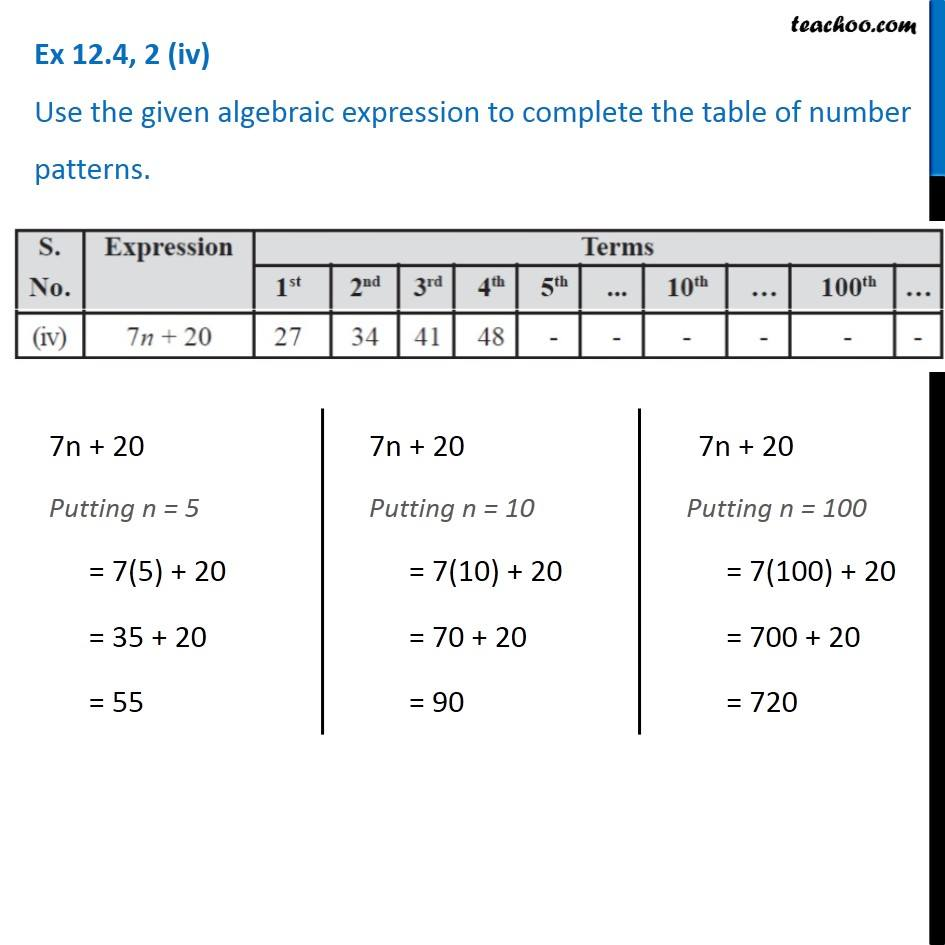Observing patterns

Chapter 10 Class 7 Algebraic Expressions
Serial order wiseLearn in your speed, with individual attention - Teachoo Maths 1-on-1 Class

### Transcript

Question 2 (iv) Use the given algebraic expression to complete the table of number patterns. 7n + 20 Putting n = 5 = 7(5) + 20 = 35 + 20 = 55 7n + 20 Putting n = 10 = 7(10) + 20 = 70 + 20 = 90 7n + 20 Putting n = 100 = 7(100) + 20 = 700 + 20 = 720